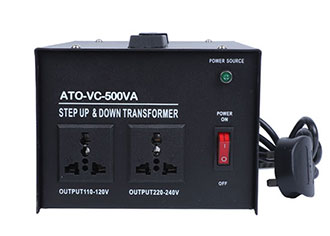# How does a Voltage Converter Work?

A voltage converter is a static electrical device that converts an AC voltage of a certain value into a voltage with the same frequency but different values. Transformers are made of two or more sets of coils wound on the same bobbin or on the same iron core.Voltage/current conversion, that is, V/I conversion, is to convert the input voltage signal into a current signal that satisfies a certain relationship. The converted current is equivalent to a constant current source with adjustable output, and its output current should be able to maintain stability without changing changes with load changes. Generally speaking, the voltage conversion circuit is realized in the form of negative feedback, which can be current series negative feedback or current parallel negative feedback, which is mainly used in industrial control and many sensor applications.

## How does the voltage converter work?

The two basic formulas for an ideal transformer are:

1. U1/U2=N1/N2, that is, for any two coils of the same transformer, the voltage is proportional to the number of turns.
2. P in = P out, that is, no matter how many secondary coils are working, the input power of the transformer is always equal to the sum of all output powers.

The principle of making a transformer: In a generator, whether the coil moves through a magnetic field or the magnetic field moves through a fixed coil, an electric potential can be induced in the coil. In both cases, the value of the magnetic flux remains unchanged, but The number of magnetic flux changes, which is the principle of mutual induction. A transformer is a device that uses electromagnetic mutual induction to transform voltage, current, and impedance.

## The composition and function of the voltage converter

The function of the voltage converter is to convert the output voltage of the multiplication and division component into a DC current. The voltage-to-current converter consists of two parts: a self-excited oscillation modulation amplifier and a power detection amplifier. The power detection amplifier composed of transistors and other components is the same as the power detection amplifier in the temperature transmitter.

## Voltage Converter Applications

In the application circuits of industrial control and many sensors, when the analog signal is output, it is usually output by voltage. When the analog signal is transmitted over a long distance in the form of voltage, the signal source resistance or the DC resistance of the transmission line will cause voltage attenuation. The lower the input resistance of the signal receiving end, the greater the voltage attenuation. In order to avoid signal attenuation during transmission, only increase the input resistance of the signal receiving end, but the increase of the input resistance of the signal receiving end reduces the anti-interference performance of the transmission line, is susceptible to external interference, and the signal transmission is unstable. For analog signals, the voltage output mode cannot be used, and the voltage output is converted into a current output. In addition, in many conventional industrial instruments, matching in the current mode also requires the output terminal to convert the voltage output into a current output. The V/I converter is to convert the voltage output signal into a current output signal, which is conducive to long-distance signal transmission. The V/I converter can be composed of various devices such as transistors.